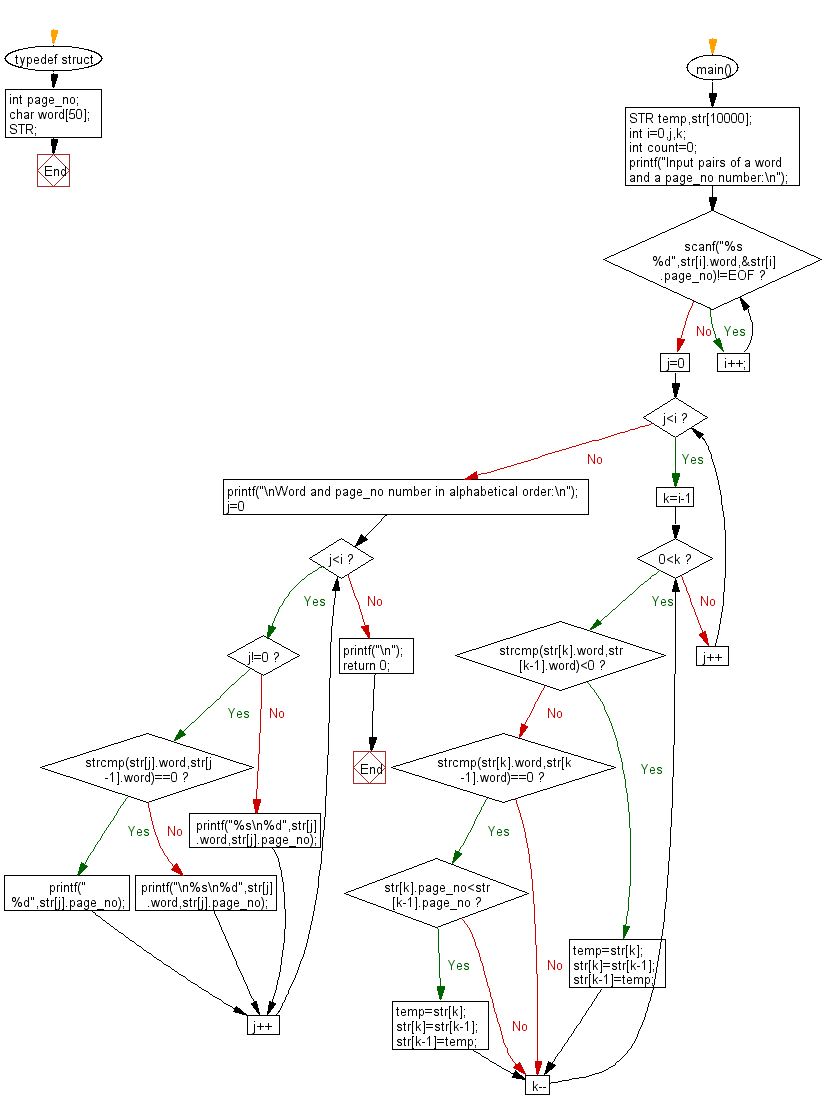﻿ C Program: Prints a word and its page number from a list

# C Exercises: Read a list of pairs of a word and a page number, and prints the word and a list of the corresponding page numbers

## C Basic Declarations and Expressions: Exercise-149 with Solution

Write a C program that reads a list of pairs of a word and a page number, and prints the word and a list of the corresponding page numbers.

The number of pairs of a word and a page number is less than or equal to 1000. A word never appears in a page more than once. The words should be printed in alphabetical order and the page numbers should be printed in ascending order.

Input:
word page_number
Output:
word
a_list_of_the_page_number
word
a_list_of_the_Page_number

Sample Solution:

C Code:

``````#include<stdio.h>
#include<string.h>

// Define a structure to hold a word and its corresponding page number
typedef struct{
int page_no;
char word;
} STR;

main(){
STR temp, str; // Declare variables of type STR
int i=0,j,k;
int count=0;

// Prompt user for input
printf("Input pairs of a word and a page_no number:\n");

// Read word and page_no pairs until end of file (EOF)
while(scanf("%s %d",str[i].word,&str[i].page_no)!=EOF){
i++;
}

// Sorting algorithm to sort words alphabetically and by page number if words are the same
for(j=0;j<i;j++){
for(k=i-1;0<k;k--){
if(strcmp(str[k].word,str[k-1].word)<0){
temp=str[k];
str[k]=str[k-1];
str[k-1]=temp;
}
else if(strcmp(str[k].word,str[k-1].word)==0){
if(str[k].page_no<str[k-1].page_no){
temp=str[k];
str[k]=str[k-1];
str[k-1]=temp;
}
}
}
}

// Print sorted word and page number pairs
printf("\nWord and page_no number in alphabetical order:\n");
for(j=0;j<i;j++){
if(j!=0){
if(strcmp(str[j].word,str[j-1].word)==0){
printf(" %d",str[j].page_no);
}
else{
printf("\n%s\n%d",str[j].word,str[j].page_no);
}
}
else{
printf("%s\n%d",str[j].word,str[j].page_no);
}
}

printf("\n");
return 0;
}
``````

Sample Output:

```Input pairs of a word and a page_no number:
Twinkle
65
Twinkle
55
Little
25
Star
35
^Z

Word and page_no number in alphabetical order:
Little
25
Star
35
Twinkle
55 65
```

Flowchart:C programming Code Editor:

What is the difficulty level of this exercise?

Test your Programming skills with w3resource's quiz.

﻿